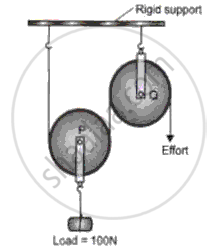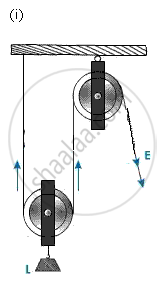Advertisement Remove all ads

# The Diagram Below Shows a Pulley Arrangement. - Physics

Answer in Brief

The diagram below shows a pulley arrangement.(i) In the diagram, mark the direction of the forces due to tension, acting on the pulley P.

(ii) What is the purpose of the pulley Q?

(iii) If the tension is T newton, deduce the relation between T and E.

(iv) Calculate the velocity ratio of the arrangement.

(v) Assuming that the efficiency of the system is 100%, what is the mechanical advantage?

(vi) Calculate the value of E

Advertisement Remove all ads

#### Solution(ii) The purpose of the pulley Q is to change the direction of application of effort to a convenient direction.

(iii) In the diagram, T = E

(iv) If the free end of the string moves, through the distance x, the load will rise by a distance x/2.

Threfore, V.R = distance moved y the effort arm / distance moved by the load = "x"/("x"//2) = 2

(v) In equilibrium,L = 2T and E = T

efficiency = M.A / V. R

or, 1 = ("M ""A")/2

⇒ M.A = 2

(vi) M.A = "L"/"E"

⇒ E = "L"/("M"."A") = 100/2 = 50 N

Is there an error in this question or solution?
Advertisement Remove all ads

#### APPEARS IN

Frank ICSE Class 10 Physics Part 2
Chapter 1 Force, Work, Energy and Power
Exercise 1.3 | Q 8 | Page 28
Advertisement Remove all ads
Advertisement Remove all ads
Share
Notifications

View all notifications

Forgot password?
Course